# Retrieve Data for Historic Analysis (SEC Method)

This web page demonstrates how to efficiently retrieve data for your financial models.  On this page I focus on how to automate acquiring data from the Securities and Exchange Commission (SEC).  I describe how to create a macro to read in the data and then clean up the data using other VBA programs and how the use the Union function. Other pages demonstrate how to use the Read pdf file to extract data into annual reports and how to read financial statement data from Market Watch into an excel file.  The Securitiy and Exchange Commission in the U.S. publishes a lot of data that can be used in corporate finance and modelling.  This data includes financial statements (10K for annual and 10Q for quarterly), acquisitions, new securities issues and investor analyst presentations.  I have made a file that automatically reads the interactive data published on the SEC website and then compile the data into a set of usable data.  Making the data usable includes a macro described below along with the UNION function. The SEC clean up file allows you to copy the data into an excel file and then it cleans up the data. The SEC clean up data file works with a macro that is somewhat like the read pdf to excel function.  To operate the clean up SEC system, you can download the file that includes the macros below. The file must be opened  after you copy data from the SEC website.  Once the file is opened, follow the instructions in the file.  At the bottom of this webpage I introduce a method to use the interactive data to make an automatic download.  The file below has the macro that you can use to clean up the data.

## Automatic Read with Interactive Data

Since 2009, you can download financial statement data directly into an excel sheet.  This is fantastic except that it is clumsy to manually download data, then put the data together from different years and then re-formatting the data.  I will work through how to create VBA code to make the process work much more quickly.  The first step to making the process more automatic is to create a macro that reads the data and puts the files into a defined folder. To see how this works, go to a page like this and right click on the red text that says “View Excel Document”.    When you right on the text named to “View Excel Document” which will find the appropriate URL.  After that copy the address and you will see a URL like the one below.  The link below is for Amazon with a code of 1018724.

https://www.sec.gov/Archives/edgar/data/1018724/000101872419000004/Financial_Report.xlsxIt is easy to put this link into workbooks open.  But the number at the end is not the same.  So instead you can use an error checking routine.  Different URLS’s for Amazon for different years are shown in the screenshot below.  Note that the final numbers are not all the same.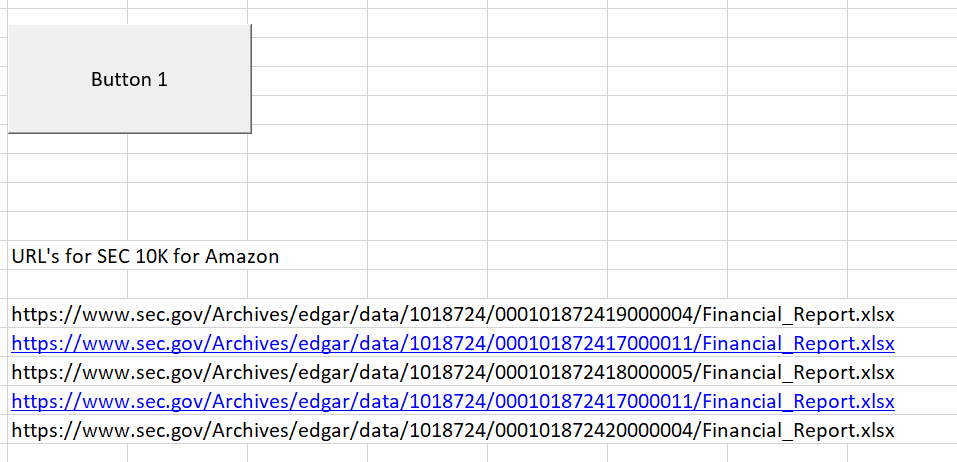Once you read in the URL’s, you can put the files together into different sheets.  I have created a macro that works through data once you have the company code number used by the SEC.  The screenshot below illustrates how you can filter through different files published by the SEC and save the files. The files are saved into an area you define.  Once you have the excel files defined, you can put the income statement, the cash flow statement and the balance sheet together.  I have made macros with short cuts (SHIFT, CNTL, I) for the income statement (SHIFT, CNTL, C) for the cash flow statement and (SHIFT, CNTL, B) for the balance sheet.  This allows you to quickly open the financial statement files and then put them together.

## Using the Union Function to Put Together Financial Statements

I have tried to make a very painful process as easy as possible.  I have not been able to make it fully automatic, but I hope you can get a file together if minutes rather than days. Like many functions, the UNION function is an array function.  This means the output does not go into one cell but rather into a series of cells.  To operate the UNION function you should leave a long space and then select the long space.  After that, you begin typing the UNION function.  Then like with any function press the tab key.  After that, you can select multiple other columns (or rows) that will be combined into one single column,  At the end press the SHIFT, CNTL, ENTER sequence.

Here is a step by step review of the union function:

### Step 2: Use the Union Function which is an array function (SHIFT, CNTL, ENTER).

Don’t forget to make enough space for a long list of columns.  The argument for the UNION function is the different titles for the stuff the you read in.  The use of the UNION function is illustrated in the screenshot below.

###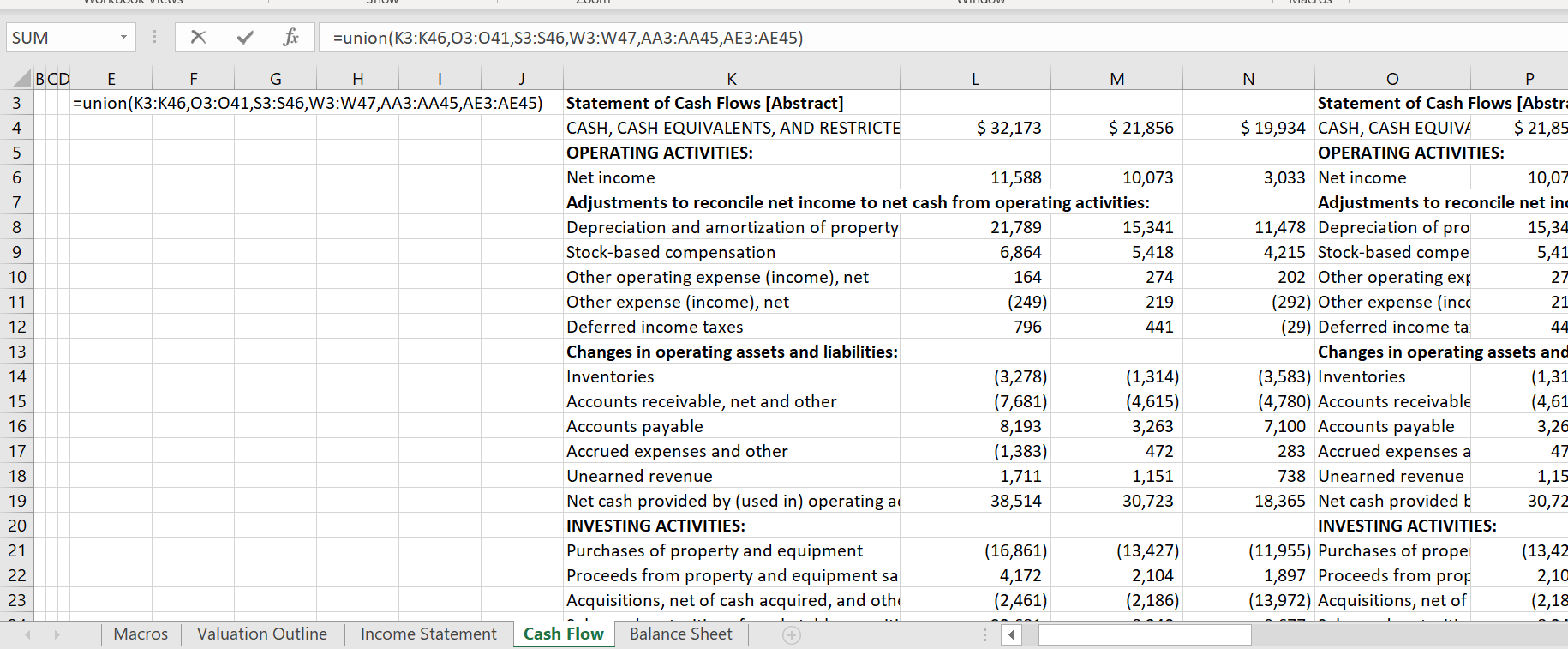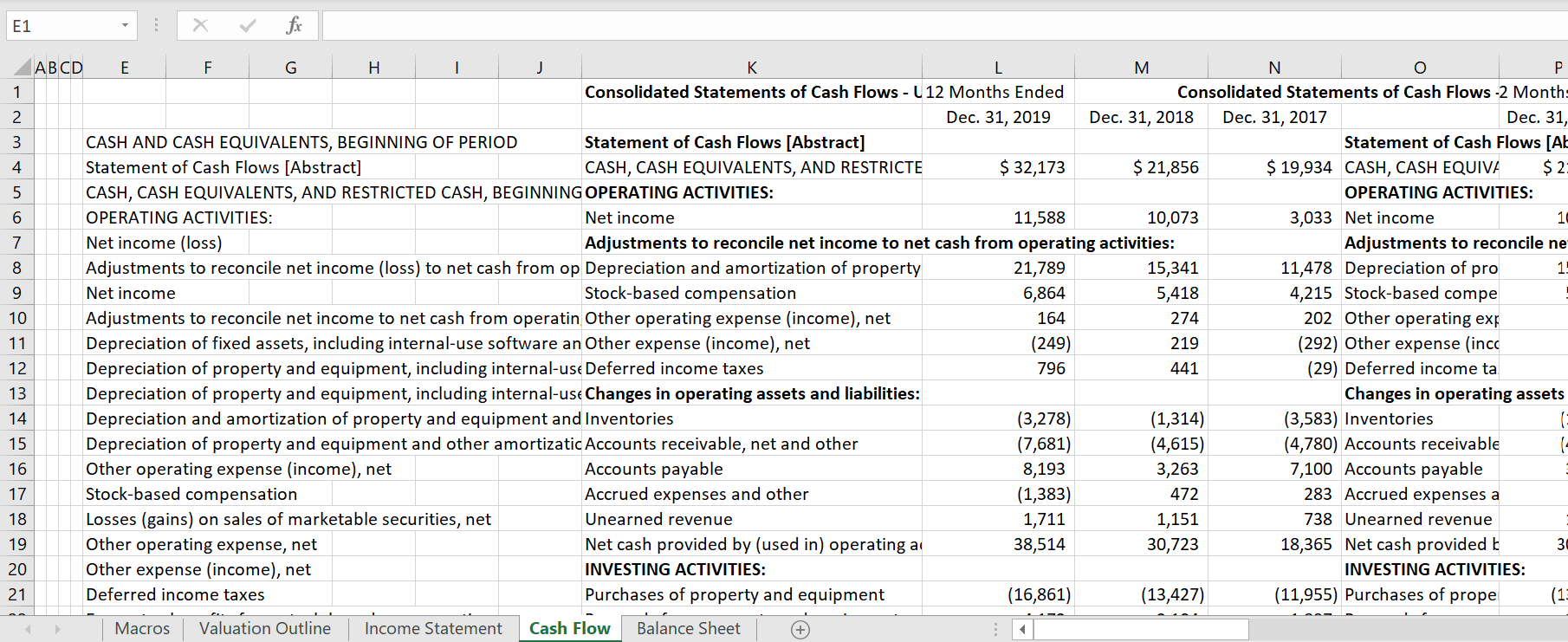### Step 3 Clean up the Union Function Output.

The union function does not always put things in a nice order.  So you can copy and paste special as values, the titles and then move then around.  The screenshot below illustrates the union function output before it has been cleaned up.  Note that there are two titles for income.  You can put these titles together and clean up the top stuff.  The second screenshot shows the cleaned up version.  Also get rid of the zeros at the end.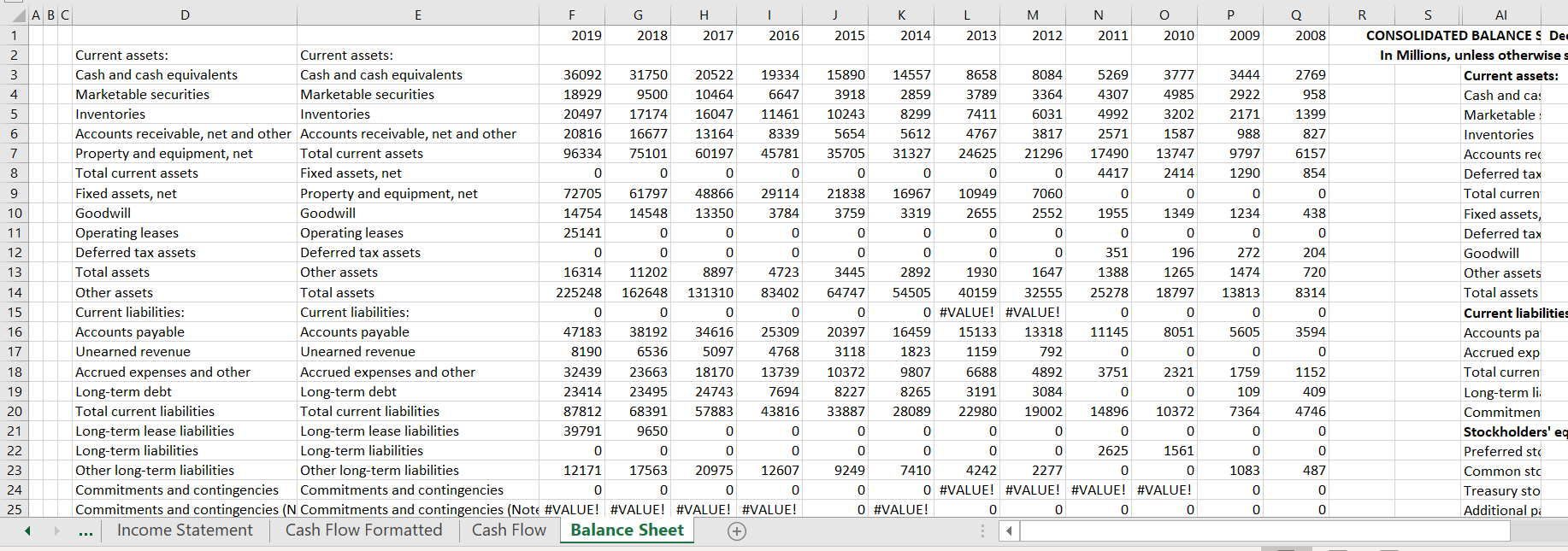### Step 4: Use the IDMAT (Index and match) to put together year by year date

You apply the INDMAT function, from the adjusted UNION function list.  You compare the master list with the year by year lists and then collect the data.  The INDMAT is illustrated in the screenshot below. When you use the INDMAT, use the entire row for the title of the individual year and use the entire rpw of data.  As you proceed with more columns you can group (temporary hide) columns that you have finished with using SHIFT, ALT, –>.  The finished result after the INDMAT is illustrated in the second screenshot below.

## Step 5: Clean Up the Data with Multiple Rows

Once you have finished with the INDMAT, it will look a bit messy as shown in the screenshot below.  But you can insert some columns (to save the original stuff for future years).  Then you can copy and paste the information to the blank columns (or even a blank sheet).  The final column will finally look clean and you have a lot of valuable history.  The result is shown in the second screenshot.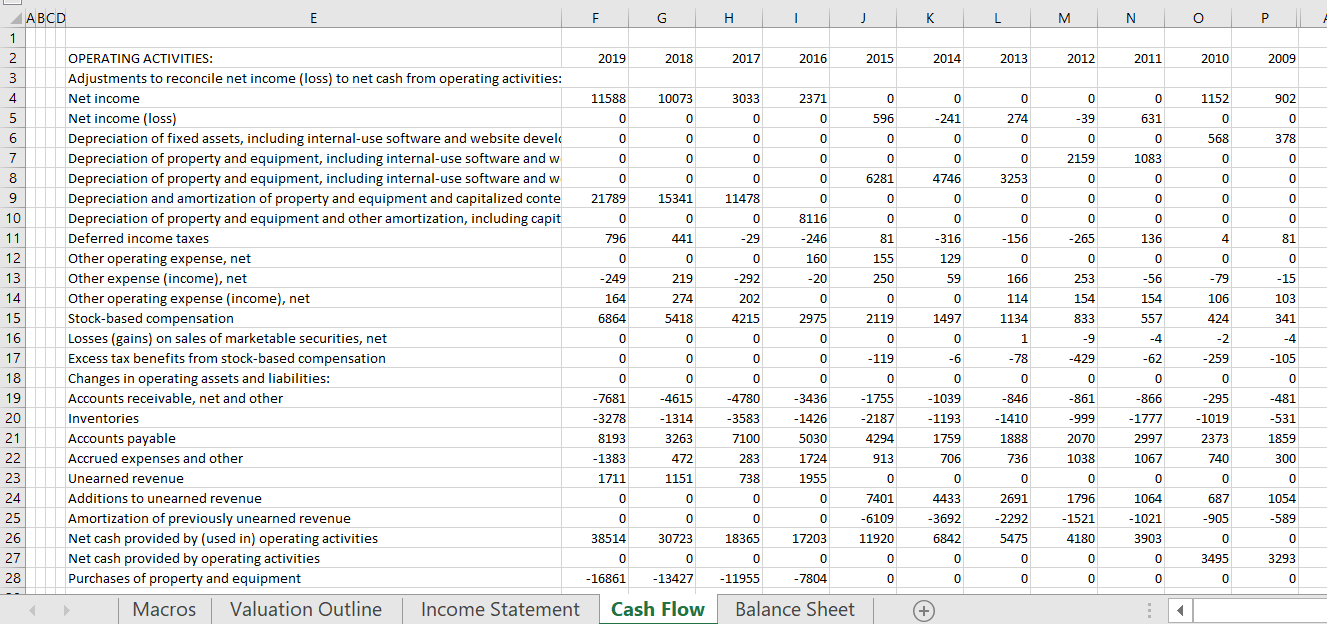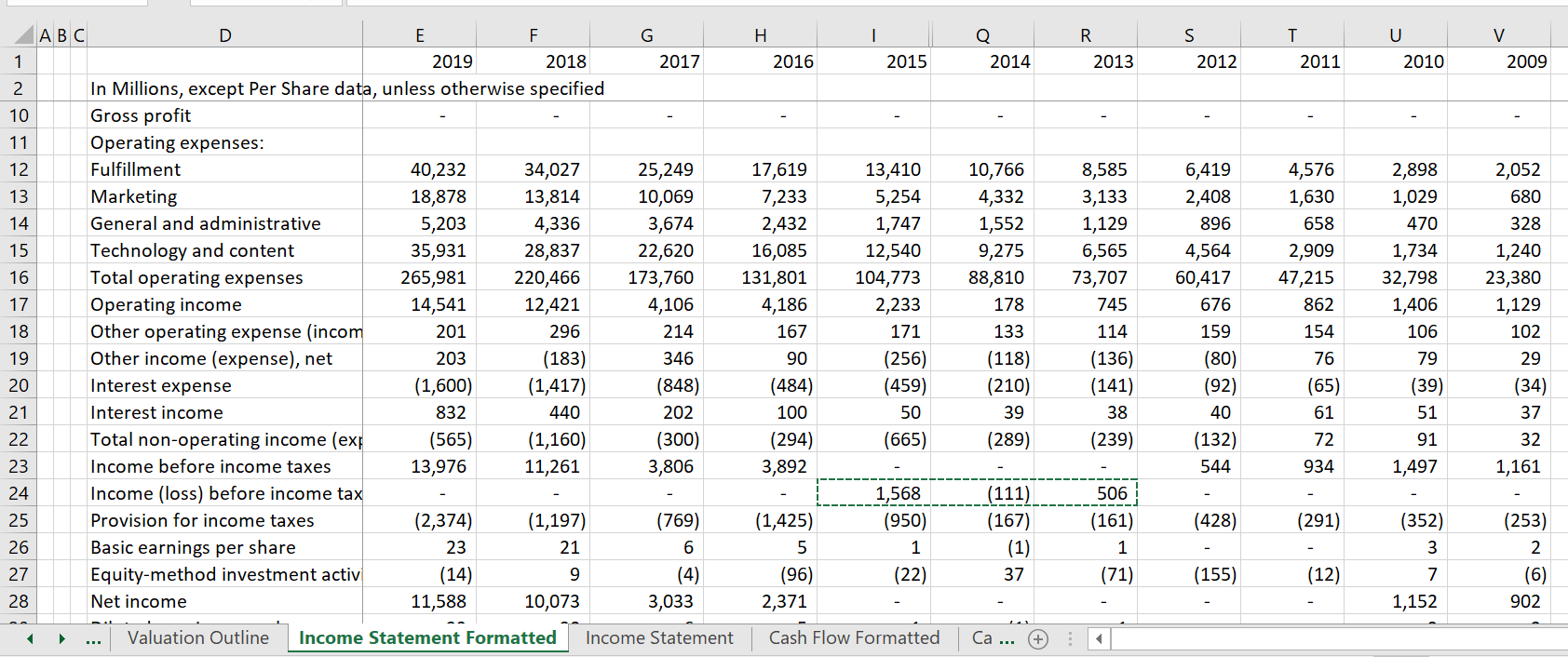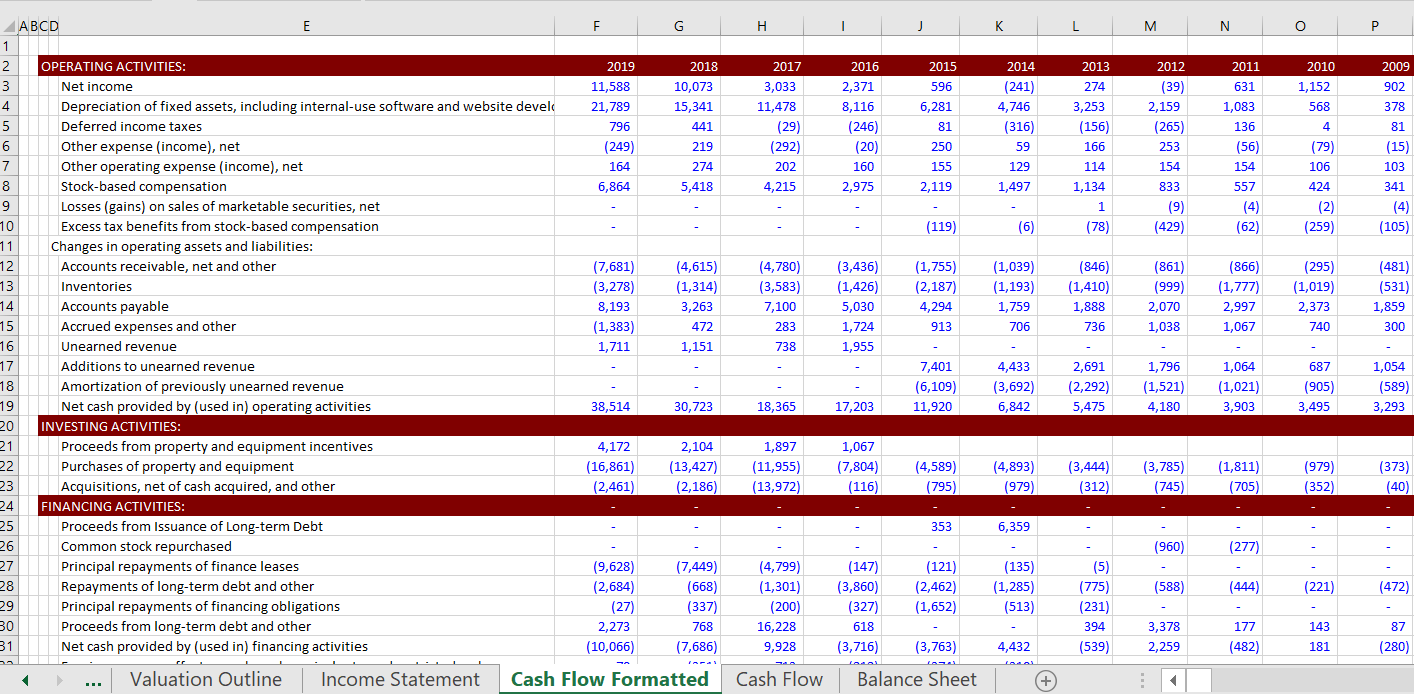The video below is lengthy and bad but it goes through this.

The second video demonstrates the VBA code that is used to create the UNION function.  This video is more technical and has various sort routined.

.

## Reading Financial HTML Data From the SEC Website and Cleaning-up the Data

Before 2009, the SEC did not publish annual reports (10-Ks) and quarterly reports (10-Qs) with its interactive button.  With these old reports, you could copy data from the HTML into a spreadsheet.  But when you copied it into a spreadsheet, it was pretty messy.  So I created a file (a little like the read pdf file) to clean up the data.  I think this is a bit obsolete now that you can get the data directly into excel.  The two videos below demonstrate how to operate the macro that cleans up the data downloaded from the SEC website.  Like any of the macros on this website, you can modify the macros yourself.

.

## Technical Details of the Union Function

The insert below shows the code for creating the UNION Function.  You can copy this function to your sheets like you can copy any UDF.  Just select the code below and copy it to a VBA module.

The code for this union function is shown in the box below:

```Function union(range1, range2, Optional range3, Optional range4, Optional range5, Optional range6, Optional range7, _
Optional range8, Optional range9, Optional range10, Optional range11, Optional range12) As Variant

Dim output(1000) As Variant ' output variables - for aggregate list
Dim output1(1000) As Variant ' variable with code number to maintain order
Dim output3(1000, 1) As Variant ' ultimate output variable

GoTo missing_test:
missing_return:

GoTo num_range: ' Get the total number for the loop through counting variables
num_range_return: ' Where to come back after getting numbers

GoTo accumulate:
accumulate_return:

' sort the output array without the numbers so that can remove duplicates

For i = 1 To tot_num

For j = i To tot_num

If output(j) < output(i) Then

str1 = output(i)
str2 = output(j)

output(i) = str2
output(j) = str1

str_1 = output1(i)
str_2 = output1(j)

output1(i) = str_2
output1(j) = str_1

End If
Next j
Next i

' eliminate duplicates from the output array without the numbers

Count = 2
For i = 2 To tot_num Step 1 ' master list

If output(Count) = output(Count - 1) Then ' if last value = prior value

For j = Count To tot_num Step 1 ' push everything up
If j > 1 Then output(j - 1) = output(j)
If j > 1 Then output1(j - 1) = output1(j)
Next j

Count = Count - 1

End If

Count = Count + 1
Next i

revised_tot = Count - 1

' re-sort with number

For i = 1 To revised_tot

For j = i To revised_tot

If output1(j) < output1(i) Then

str1 = output1(i)
str2 = output1(j)

output1(i) = str2
output1(j) = str1

str_1 = output(i)
str_2 = output(j)

output(i) = str_2
output(j) = str_1

End If

Next j

Next i

For i = 1 To revised_tot
output3(i, 1) = output(i)
Next i

union = output3
Exit Function

missing_test:

' Create test for optional variables when you enter a variable

range3_option = True
range4_option = True
range5_option = True
range6_option = True
range7_option = True
range8_option = True
range9_option = True
range10_option = True
range11_option = True
range12_option = True

If IsMissing(range3) Then range3_option = False
If IsMissing(range4) Then range4_option = False
If IsMissing(range5) Then range5_option = False
If IsMissing(range6) Then range6_option = False
If IsMissing(range7) Then range7_option = False
If IsMissing(range8) Then range8_option = False
If IsMissing(range9) Then range9_option = False
If IsMissing(range10) Then range10_option = False
If IsMissing(range11) Then range11_option = False
If IsMissing(range12) Then range12_option = False

GoTo missing_return:

' count the number in the series

num_range:

num1 = range1.Count
num2 = range2.Count

If range3_option Then num3 = range3.Count
If range4_option Then num4 = range4.Count
If range5_option Then num5 = range5.Count
If range6_option Then num6 = range6.Count
If range7_option Then num7 = range7.Count
If range8_option Then num8 = range8.Count
If range9_option Then num9 = range9.Count
If range10_option Then num10 = range10.Count
If range11_option Then num11 = range11.Count
If range12_option Then num12 = range12.Count

tot_num = num1 + num2 + num3 + num4 + num5 + num6 + num7 + num8 + num9 + num10 + num11 + num12
revised_tot = tot_num

GoTo num_range_return:

accumulate:

' Accumulate the data into output and output1

For i = 1 To num1 ' accumulate into a single array
Count = Count + 1

output1(Count) = i + 100 & " " & range1(i) ' use code numbers to maintain the order of the numbers
output(Count) = range1(i) ' make a big array with all of the ranges

Next i

For i = 1 To num2
Count = Count + 1

output1(Count) = i + 100 & " " & range2(i)
output(Count) = range2(i)

Next i

If range3_option Then
For i = 1 To num3
Count = Count + 1

output1(Count) = i + 100 & " " & range3(i)
output(Count) = range3(i)
Next i
End If

If range4_option Then ' only do when passed the missing test for range
For i = 1 To num4 ' accumulate into an aggregate single array
Count = Count + 1

output1(Count) = i + 100 & " " & range4(i) ' use code numbers to maintain the order of the numbers
output(Count) = range4(i) ' make a big array with all of the ranges

Next i
End If ' end of missing test on range

If range5_option Then ' only do when passed the missing test for range
For i = 1 To num5 ' accumulate into an aggregate single array
Count = Count + 1

output1(Count) = i + 100 & " " & range5(i) ' use code numbers to maintain the order of the numbers
output(Count) = range5(i) ' make a big array with all of the ranges

Next i
End If ' end of missing test on range

If range6_option Then ' only do when passed the missing test for range
For i = 1 To num6 ' accumulate into an aggregate single array
Count = Count + 1

output1(Count) = i + 100 & " " & range6(i) ' use code numbers to maintain the order of the numbers
output(Count) = range6(i) ' make a big array with all of the ranges

Next i
End If ' end of missing test on range

If range7_option Then ' only do when passed the missing test for range
For i = 1 To num7 ' accumulate into an aggregate single array
Count = Count + 1

output1(Count) = i + 100 & " " & range7(i) ' use code numbers to maintain the order of the numbers
output(Count) = range7(i) ' make a big array with all of the ranges

Next i
End If ' end of missing test on range

If range8_option Then ' only do when passed the missing test for range
For i = 1 To num8 ' accumulate into an aggregate single array
Count = Count + 1

output1(Count) = i + 100 & " " & range8(i) ' use code numbers to maintain the order of the numbers
output(Count) = range8(i) ' make a big array with all of the ranges

Next i
End If ' end of missing test on range

If range9_option Then ' only do when passed the missing test for range
For i = 1 To num9 ' accumulate into an aggregate single array
Count = Count + 1

output1(Count) = i + 100 & " " & range9(i) ' use code numbers to maintain the order of the numbers
output(Count) = range9(i) ' make a big array with all of the ranges

Next i
End If ' end of missing test on range

If range10_option Then ' only do when passed the missing test for range
For i = 1 To num10 ' accumulate into an aggregate single array
Count = Count + 1

output1(Count) = i + 100 & " " & range10(i) ' use code numbers to maintain the order of the numbers
output(Count) = range10(i) ' make a big array with all of the ranges

Next i
End If ' end of missing test on range

If range11_option Then ' only do when passed the missing test for range
For i = 1 To num11 ' accumulate into an aggregate single array
Count = Count + 1

output1(Count) = i + 100 & " " & range11(i) ' use code numbers to maintain the order of the numbers
output(Count) = range11(i) ' make a big array with all of the ranges

Next i
End If ' end of missing test on range

If range12_option Then ' only do when passed the missing test for range
For i = 1 To num12 ' accumulate into an aggregate single array
Count = Count + 1

output1(Count) = i + 100 & " " & range12(i) ' use code numbers to maintain the order of the numbers
output(Count) = range12(i) ' make a big array with all of the ranges

Next i
End If ' end of missing test on range

tot_num = Count
revised_tot = tot_num

GoTo accumulate_return:

End Function

Sub A_union_function_help()

UserForm11.Show

GoTo skip
MsgBox _
Chr(1) & Chr(2) & Chr(3) & Chr(4) & Chr(5) & Chr(6) & Chr(7) & Chr(8) & Chr(9) & Chr(10) & Chr(11) & " Test Chars " & Chr(13) & Chr(13) & Chr(13) & _
Chr(11) & Chr(12) & Chr(13) & Chr(14) & Chr(15) & Chr(16) & Chr(17) & Chr(18) & Chr(19) & Chr(20) & Chr(21) & " Find the A_function_help macro and copy entire module to you workbook" & Chr(13) & Chr(13) & Chr(13) & _
Chr(21) & Chr(22) & Chr(23) & Chr(24) & Chr(25) & Chr(26) & Chr(27) & Chr(28) & Chr(29) & Chr(30) & Chr(31) & " Find the A_function_help macro and copy entire module to you workbook" & Chr(13) & Chr(13) & Chr(13) & _
" Please make sure the Option Base 1 is at the top " & Chr(13) & Chr(13) & Chr(13) & _
" To Sort, for now you must copy and paste special " & Chr(13) & Chr(13) & Chr(13) & _
" There is an INDMAT -- Index and Match Function. This can be used with entire columns or rows" & Chr(13) & Chr(13) & Chr(13)

skip:

MsgBox _
" Find the UNION functions (See the Button Below). COPY entire module to you workbook" & Chr(13) & Chr(13) & Chr(13) & _
" Make sure the Option Base 1 is at the top " & Chr(13) & Chr(13) & Chr(13) & _
" Use the UNION function to get a TITLE List -- Make Similar Titles " & Chr(13) & Chr(13) & Chr(13) & _
" The UNION is only for getting a good master list of Similar Titles " & Chr(13) & Chr(13) & Chr(13) & _
" Use the INDMAT function for the Numbers -- Enter the Account Title, the Master Title and the Numbers " & Chr(13) & Chr(13) & Chr(13) & _
" To Sort, for now you must copy and paste special " & Chr(13) & Chr(13) & Chr(13) & _
" The INDMAT -- Index and Match Function makes things easier. This can be used with entire columns or rows" & Chr(13) & Chr(13) & Chr(13), , _
" MAKING THE UNION AND INDMAT WORK FOR READING FINANCIALS"

MsgBox _
" After Reading in the Financials, you should do some PREPARATION " & Chr(13) & Chr(13) & Chr(13) & _
" 1. Put the Financials in the Same Sheet " & Chr(13) & Chr(13) & Chr(13) & _
" 2. Put the years in Order " & Chr(13) & Chr(13) & Chr(13) & _
" 3. Adjust Titles to Make Them Similar " & Chr(13) & Chr(13) & Chr(13) & _
" 4. Apply the UNION function for a comprehensive set of titles " & Chr(13) & Chr(13) & Chr(13) & _
" 5. Use the INDMAT function with locked columns and copy down " & Chr(13) & Chr(13) & Chr(13) & _
" The INDMAT -- Index and Match Function makes things easier. This can be used with entire columns or rows" & Chr(13) & Chr(13) & Chr(13), , _
" MAKING THE UNION AND INDMAT WORK FOR READING FINANCIALS"

End Sub

```

## Technical Details of the INDMAT

The insert below shows the code for creating the INDMAT Function.  You can copy this function to your sheets like for the other functions.

```Function indmat(lookup_value, master_list, values) As Single ' This function does the index and match together

Dim num As Single
num = 150

For i = 1 To num ' loop around a given number
If lookup_value = master_list(i) Then
indmat = values(i)
Exit Function
End If
Next i

' On Error GoTo no_value:
' match1 = WorksheetFunction.Match(lookup_value, master_list, 0)
' indmat = WorksheetFunction.Index(values, match1)

' Exit Function
no_value:
indmat = 0

End Function

```